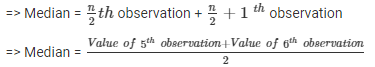Courses

# Test: Measures Of Central Tendency

## 10 Questions MCQ Test Mathematics (Maths) Class 9 | Test: Measures Of Central Tendency

Description
This mock test of Test: Measures Of Central Tendency for Class 9 helps you for every Class 9 entrance exam. This contains 10 Multiple Choice Questions for Class 9 Test: Measures Of Central Tendency (mcq) to study with solutions a complete question bank. The solved questions answers in this Test: Measures Of Central Tendency quiz give you a good mix of easy questions and tough questions. Class 9 students definitely take this Test: Measures Of Central Tendency exercise for a better result in the exam. You can find other Test: Measures Of Central Tendency extra questions, long questions & short questions for Class 9 on EduRev as well by searching above.
QUESTION: 1

### The mean of prime numbers between 20 and 30 is:

Solution:

The prime numbers between 20 and 30 are 23, 29.
► Mean of 23 and 29 = (23 + 29) / 2 = 52/ 2 = 26

QUESTION: 2

### The arithmetic mean of 30 values is 69. The new mean, if each of the 30 values is increased by 5 is:

Solution:

Mean=30 then total sum=30×69=2070 if 5 increase to all then total sum=2070+30×5=2070+150=2220
New mean =2220÷30 =74

QUESTION: 3

### In an examination, ten students scored the following marks: 60, 58, 90, 51, 47, 81, 70, 95, 87, 99. The range of this data is:

Solution:

The difference between the maximum & minimum value of the observation is called as range.
So Range = 99-47 = 52

QUESTION: 4

If the number of observations in a data set is 25 and the mean of the data is 32. Then, the sum of all observations is:

Solution:

The number of observation is 25. The mean is 32.
► The sum = 25 + 25 + 25... 32 times
► 32*25 = 800

QUESTION: 5

If the mean of 6, 4, 7, x and 10 is 8, the value of x is:

Solution:

Mean = sum of all obs. /no. of obs.
► 8 = (6+4+7+x+10)/5
► 40 = 27+x
► x = 13

QUESTION: 6

The maximum frequency is 10 for observation 4. Hence the mode of the data is:

Solution:
• The mode is the most frequently occurring value of data.
• Here 4 is the most frequently occurring observation. So 4 is the mode of the data.
QUESTION: 7

Median of the data 5, 9, 8, 6, 3, 5, 7, 12, 15 is:

Solution:

Arranging the given terms in descending order 15, 12, 9, 8, 7, 6, 5, 5, 3
► Number of terms = 9
► Median = (n+1)/2 = 10/2 = 5th term = 7

QUESTION: 8

Find median of following data: 83, 37, 70, 29, 45, 63, 41, 70, 34, 54:

Solution:

Arranging the data in ascending order, we have:
29, 34, 37, 41, 45, 54, 63, 70, 70, 83
Here, the number of observations, n = 10 (Even).Hence, the median of the given data is 49.5.

QUESTION: 9

What will be the mean of x, x + 2, x + 4, x + 6, x + 8, when the value of x is 12?

Solution:

► Mean = sum of all the obs./ no. of obs.
In the given question number of observation is 5.
► Sum of all observations = 5x + 20
But x =12; on substituting the value of x we get 5 * 12 + 20 = 80.
► Mean = 80 / 5 = 16

QUESTION: 10

The median of observations 17, 18, 20, 22, x+2, x+4, 31, 35, 37 is found to be 24. If the observations have been arranged in ascending order, the value of x will be​:

Solution:

17, 18, 20, 22, x+2, x+4, 31, 35, 37
The total number of observation is 9, which is odd.
► Median  = (9+1)/2th term = 5th term
Now 5th term of the observation is (x+2)
Also given, median = 24
► x + 2 = 24
► x = 24-2
► x = 22
So the value of x is 22.